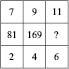# User Forum

Subject :IMO    Class : Class 5

There is a matrix that follows a certain rule row-wise or column-wise. Find the missing number.(a) 212
(b) 241
(c) 144
(d) 289

D

## Ans 1:

Class : Class 4
Letâs see what pattern is being followed by the numbers in the above grid. Letâs take the first column â 7, 81, 2 If we add 1st and 3rd number = 7 2 = 9 And if we multiply 9 by 9 itself = 9 x 9 = 81. 81 is the 2nd number in the first column. Letâs see if the numbers in second column follow the same pattern. 9, 169, 4 We add 1st and 3rd number = 9 4 = 13 We multiply 13 by 13 itself = 13 x 13 = 169. 169 is the 2nd number in the second column. That means the numbers of third column will follow the same pattern. 11, ?, 6 We add 1st and 3rd number = 11 6 = 17 We multiply 17 by 17 itself = 17 x 17 = 289. 289 will be the missing number in the third column. So, the correct answer is Option D.

Class : Class 8
D

## Ans 3:

Class : Class 6
It is D because 11 6=17and 17x17 is 289.